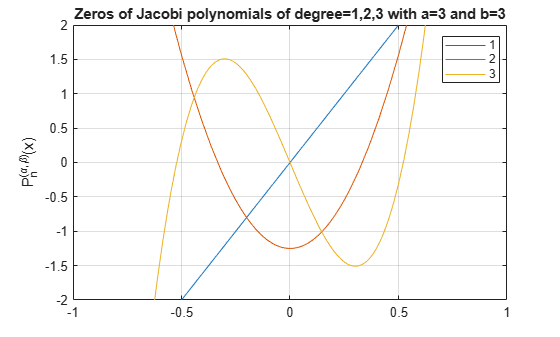# jacobiP

Jacobi polynomials

## Syntax

jacobiP(n,a,b,x)

## Description

example

jacobiP(n,a,b,x) returns the nth degree Jacobi polynomial with parameters a and b at x.

## Examples

### Find Jacobi Polynomials for Numeric and Symbolic Inputs

Find the Jacobi polynomial of degree 2 for numeric inputs.

jacobiP(2,0.5,-3,6)
ans = 7.3438

Find the Jacobi polynomial for symbolic inputs.

syms n a b x jacobiP(n,a,b,x)
ans = jacobiP(n, a, b, x)

If the degree of the Jacobi polynomial is not specified, jacobiP cannot find the polynomial and returns the function call.

Specify the degree of the Jacobi polynomial as 1 to return the form of the polynomial.

J = jacobiP(1,a,b,x)
J = a/2 - b/2 + x*(a/2 + b/2 + 1)

To find the numeric value of a Jacobi polynomial, call jacobiP with the numeric values directly. Do not substitute into the symbolic polynomial because the result can be inaccurate due to round-off. Test this by using subs to substitute into the symbolic polynomial, and compare the result with a numeric call.

J = jacobiP(300, -1/2, -1/2, x); subs(J,x,vpa(1/2)) jacobiP(300, -1/2, -1/2, vpa(1/2))
ans = 101573673381249394050.64541318209 ans = 0.032559931334979678350422392588404

When subs is used to substitute into the symbolic polynomial, the numeric result is subject to round-off error. The direct numerical call to jacobiP is accurate.

### Find Jacobi Polynomial with Vector and Matrix Inputs

Find the Jacobi polynomials of degrees 1 and 2 by setting n = [1 2] for a = 3 and b = 1.

syms x jacobiP([1 2],3,1,x)
ans = [ 3*x + 1, 7*x^2 + (7*x)/2 - 1/2]

jacobiP acts on n element-wise to return a vector with two entries.

If multiple inputs are specified as a vector, matrix, or multidimensional array, these inputs must be the same size. Find the Jacobi polynomials for a = [1 2;3 1], b = [2 2;1 3], n = 1 and x.

a = [1 2;3 1]; b = [2 2;1 3]; J = jacobiP(1,a,b,x)
J = [ (5*x)/2 - 1/2, 3*x] [ 3*x + 1, 3*x - 1]

jacobiP acts element-wise on a and b to return a matrix of the same size as a and b.

### Visualize Zeros of Jacobi Polynomials

Plot Jacobi polynomials of degree 1, 2, and 3 for a = 3, b = 3, and -1<x<1. To better view the plot, set axis limits by using axis.

syms x fplot(jacobiP(1:3,3,3,x)) axis([-1 1 -2 2]) grid on ylabel('P_n^{(\alpha,\beta)}(x)') title('Zeros of Jacobi polynomials of degree=1,2,3 with a=3 and b=3'); legend('1','2','3','Location','best')### Prove Orthogonality of Jacobi Polynomials with Respect to Weight Function

The Jacobi polynomials P(n,a,b,x) are orthogonal with respect to the weight function ${\left(1-x\right)}^{a}{\left(1-x\right)}^{b}$ on the interval [-1,1].

Prove P(3,a,b,x) and P(5,a,b,x) are orthogonal with respect to the weight function ${\left(1-x\right)}^{a}{\left(1-x\right)}^{b}$ by integrating their product over the interval [-1,1], where a = 3.5 and b = 7.2.

syms x a = 3.5; b = 7.2; P3 = jacobiP(3, a, b, x); P5 = jacobiP(5, a, b, x); w = (1-x)^a*(1+x)^b; int(P3*P5*w, x, -1, 1)
ans = 0

## Input Arguments

collapse all

Degree of Jacobi polynomial, specified as a nonnegative integer, or a vector, matrix, or multidimensional array of nonnegative integers, or a symbolic nonnegative integer, variable, vector, matrix, function, expression, or multidimensional array.

Input, specified as a number, vector, matrix, multidimensional array, or a symbolic number, vector, matrix, function, expression, or multidimensional array.

Input, specified as a number, vector, matrix, multidimensional array, or a symbolic number, vector, matrix, function, expression, or multidimensional array.

Evaluation point, specified as a number, vector, matrix, multidimensional array, or a symbolic number, vector, matrix, function, expression, or multidimensional array.

collapse all

### Jacobi Polynomials

• The Jacobi polynomials are given by the recursion formula

$\begin{array}{l}2n{c}_{n}{c}_{2n-2}P\left(n,a,b,x\right)={c}_{2n-1}\left({c}_{2n-2}{c}_{2n}x+{a}^{2}-{b}^{2}\right)P\left(n-1,a,b,x\right)\\ \text{ }\text{ }\text{ }\text{ }\text{ }\text{ }\text{ }\text{ }-2\left(n-1+a\right)\left(n-1+b\right){c}_{2n}P\left(n-2,a,b,x\right),\\ \text{where}\\ {c}_{n}=n+a+b\\ P\left(0,a,b,x\right)=1\\ P\left(1,a,b,x\right)=\frac{a-b}{2}+\left(1+\frac{a+b}{2}\right)x.\end{array}$
• For fixed real a > -1 and b > -1, the Jacobi polynomials are orthogonal on the interval [-1,1] with respect to the weight function $w\left(x\right)={\left(1-x\right)}^{a}{\left(1+x\right)}^{b}$.

• For a = 0 and b = 0, the Jacobi polynomials P(n,0,0,x) reduce to the Legendre polynomials P(n, x).

• The relation between Jacobi polynomials P(n,a,b,x) and Chebyshev polynomials of the first kind T(n,x) is

$T\left(n,x\right)=\frac{{2}^{2n}{\left(n!\right)}^{2}}{\left(2n\right)!}P\left(n,-\frac{1}{2},-\frac{1}{2},x\right).$
• The relation between Jacobi polynomials P(n,a,b,x) and Chebyshev polynomials of the second kind U(n,x) is

$U\left(n,x\right)=\frac{{2}^{2n}n!\left(n+1\right)!}{\left(2n+1\right)!}P\left(n,\frac{1}{2},\frac{1}{2},x\right).$
• The relation between Jacobi polynomials P(n,a,b,x) and Gegenbauer polynomials G(n,a,x) is

$G\left(n,a,x\right)=\frac{\Gamma \left(a+\frac{1}{2}\right)\Gamma \left(n+2a\right)}{\Gamma \left(2a\right)\Gamma \left(n+a+\frac{1}{2}\right)}P\left(n,a-\frac{1}{2},a-\frac{1}{2},x\right).$

## Version History

Introduced in R2014b